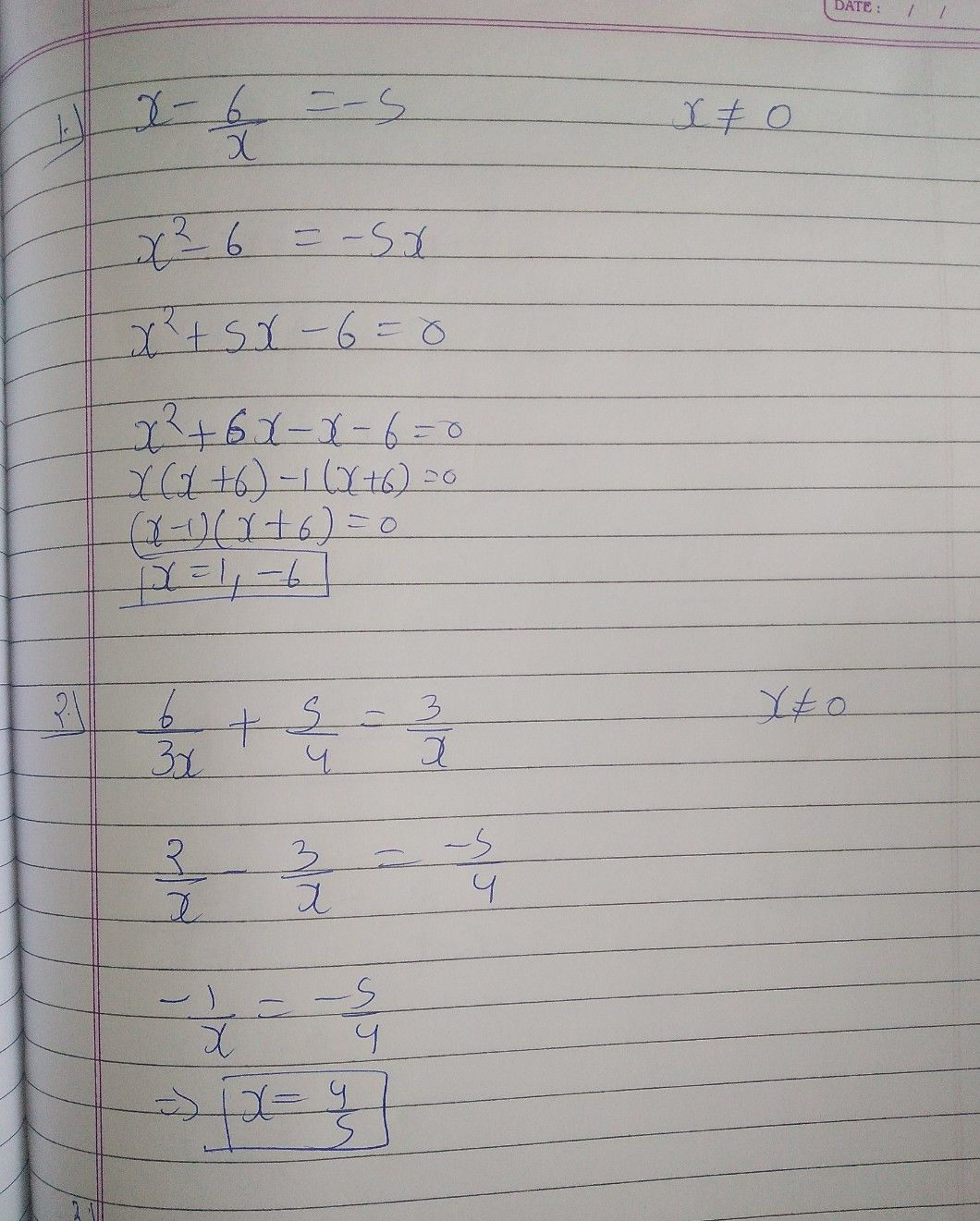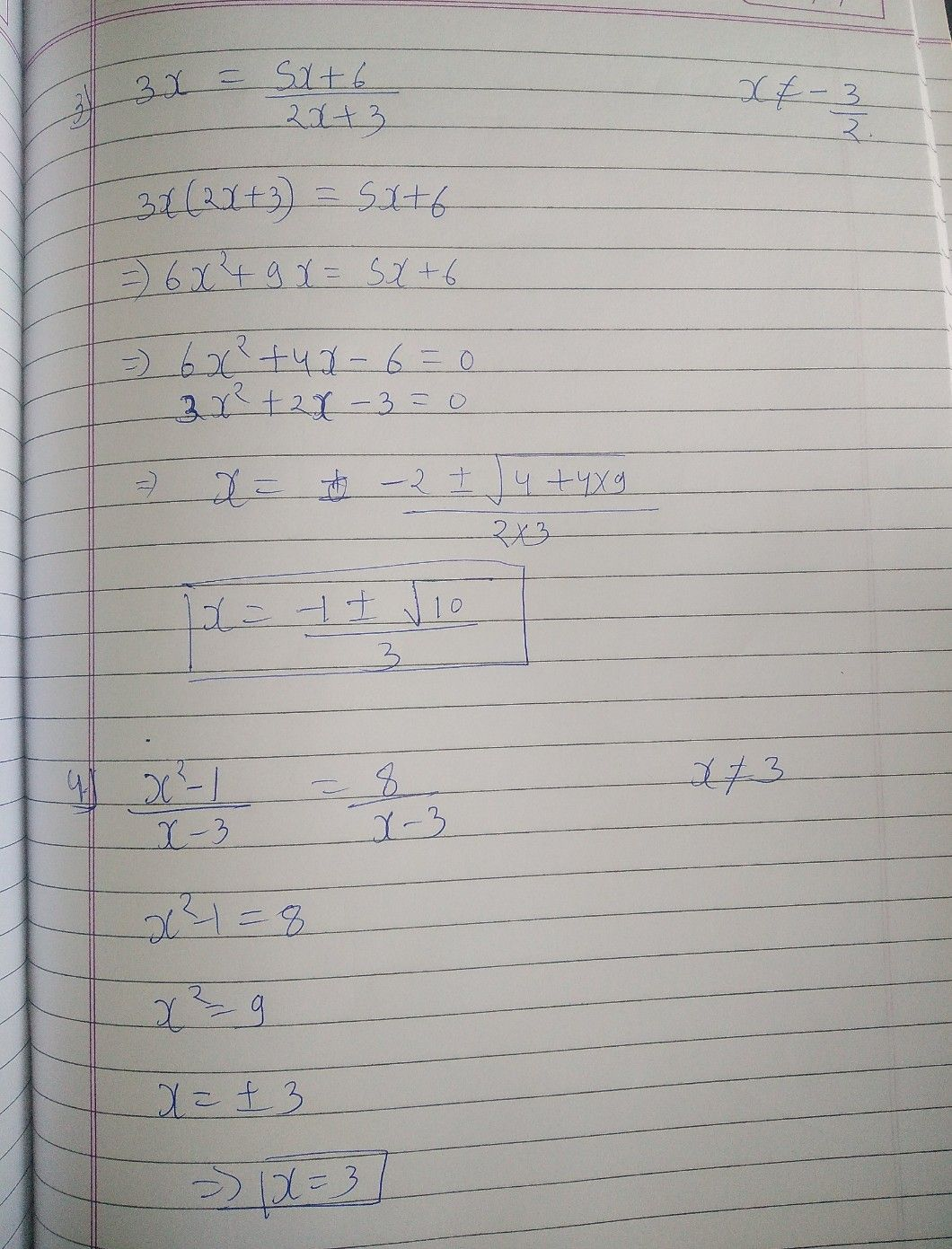Symbol
ProblemActivity A. Solve the following rational equations. Check for extraneous solution. $1.$ $6$ $x--=-5$ $\dfrac {6} {x}=-5$ $3.3x=\dfrac {5x+6} {2x+3}$ $2.$ $\dfrac {6} {3x}+\dfrac {5} {4}=\dfrac {3} {x}$ $4.3$ $\dfrac {x^{2}-1} {x-3}=\dfrac {8} {x-3}$
10th-13th grade
Other
Search count: 106
SolutionQanda teacher - MeenaIf you have any doubt in given problem feel free to ask:) Dear, Please evaluate the answer and give gift coins(it does not reduce your coin balance.) for giving gift coins Click on evaluate. Then press the + icon 3 times(3000) (it does not reduce your coin balance)